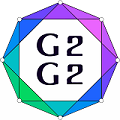#Graphs and Groups, Geometries and GAP (G2G2) Summer School - External Satellite Conference of 8ECM

27 June 2021 to 3 July 2021
Rogla, Slovenia
UTC timezone

## On maximal cliques in Paley graphs of square order

Not scheduled
20m
Rogla, Slovenia

#### Rogla, Slovenia

Hotel Planja, Rogla 1, 3214 Zreče, Slovenia
Oral presentation

### Speaker

Dr Sergey Goryainov (Chelyabinsk State University)

### Description

In , Blokhuis studied maximum cliques in Paley graphs of square order $𝑃(𝑞^2)$. It was shown that a clique of size $q$ in $𝑃(𝑞^2)$ is necessarily a quadratic line in the corresponding affine plane $𝐴(2,𝑞)$.

Let $𝑟(𝑞)$ denote the reminder after division of $𝑞$ by $4$. In , for any odd prime power $𝑞$, a maximal (but not maximum) clique in $𝑃(𝑞^2)$ of size $\frac{𝑞+𝑟(𝑞)}{2}$ was constructed.

In , for any odd prime power $𝑞$, a maximal clique in $𝑃(𝑞^2)$ of the same size $\frac{𝑞+𝑟(𝑞)}{2}$ was constructed. This clique was shown to have a remarkable connection with eigenfunctions of $𝑃(𝑞^2)$ that have minimum cardinality of support $𝑞+1$.

In this talk, we discuss the constructions of maximal cliques from  and  and establish a correspondence between them.

Acknowledgments. Sergey Goryainov and Leonid Shalaginov are supported by RFBR according to the research project 20-51-53023.

References
 A. Blokhuis, On subsets of $𝐺𝐹(𝑞^2)$ with square differences. Indag. Math. 46 (1984) 369–372.
 R. D. Baker, G. L. Ebert, J. Hemmeter, A. J. Woldar, Maximal cliques in the Paley graph of square order. J. Statist. Plann. Inference 56 (1996) 33–38.
 S. V. Goryainov, V. V. Kabanov, L. V. Shalaginov, A. A. Valyuzhenich, On eigenfunctions and maximal cliques of Paley graphs of square order. Finite Fields and Their Applications 52 (2018) 361–369.
 S. V. Goryainov, A. V. Masley, L. V. Shalaginov, On a correspondence between maximal cliques in Paley graphs of square order. arXiv:2102.03822 (2021).

### Primary author

Dr Sergey Goryainov (Chelyabinsk State University)